» Code examples / Computer Vision / Classification using Attention-based Deep Multiple Instance Learning (MIL).

# Classification using Attention-based Deep Multiple Instance Learning (MIL).

Date created: 2021/08/16
Description: MIL approach to classify bags of instances and get their individual instance score.View in ColabGitHub source

## Introduction

### What is Multiple Instance Learning (MIL)?

Usually, with supervised learning algorithms, the learner receives labels for a set of instances. In the case of MIL, the learner receives labels for a set of bags, each of which contains a set of instances. The bag is labeled positive if it contains at least one positive instance, and negative if it does not contain any.

### Motivation

It is often assumed in image classification tasks that each image clearly represents a class label. In medical imaging (e.g. computational pathology, etc.) an entire image is represented by a single class label (cancerous/non-cancerous) or a region of interest could be given. However, one will be interested in knowing which patterns in the image is actually causing it to belong to that class. In this context, the image(s) will be divided and the subimages will form the bag of instances.

Therefore, the goals are to:

1. Learn a model to predict a class label for a bag of instances.
2. Find out which instances within the bag caused a position class label prediction.

### Implementation

The following steps describe how the model works:

1. The feature extractor layers extract feature embeddings.
2. The embeddings are fed into the MIL attention layer to get the attention scores. The layer is designed as permutation-invariant.
3. Input features and their corresponding attention scores are multiplied together.
4. The resulting output is passed to a softmax function for classification.

## Setup

``````import numpy as np
import tensorflow as tf
from tensorflow import keras
from tensorflow.keras import layers
from tqdm import tqdm
from matplotlib import pyplot as plt

plt.style.use("ggplot")
``````

## Create dataset

We will create a set of bags and assign their labels according to their contents. If at least one positive instance is available in a bag, the bag is considered as a positive bag. If it does not contain any positive instance, the bag will be considered as negative.

### Configuration parameters

• `POSITIVE_CLASS`: The desired class to be kept in the positive bag.
• `BAG_COUNT`: The number of training bags.
• `VAL_BAG_COUNT`: The number of validation bags.
• `BAG_SIZE`: The number of instances in a bag.
• `PLOT_SIZE`: The number of bags to plot.
• `ENSEMBLE_AVG_COUNT`: The number of models to create and average together. (Optional: often results in better performance - set to 1 for single model)
``````POSITIVE_CLASS = 1
BAG_COUNT = 1000
VAL_BAG_COUNT = 300
BAG_SIZE = 3
PLOT_SIZE = 3
ENSEMBLE_AVG_COUNT = 1
``````

### Prepare bags

Since the attention operator is a permutation-invariant operator, an instance with a positive class label is randomly placed among the instances in the positive bag.

``````def create_bags(input_data, input_labels, positive_class, bag_count, instance_count):

# Set up bags.
bags = []
bag_labels = []

# Normalize input data.
input_data = np.divide(input_data, 255.0)

# Count positive samples.
count = 0

for _ in range(bag_count):

# Pick a fixed size random subset of samples.
index = np.random.choice(input_data.shape, instance_count, replace=False)
instances_data = input_data[index]
instances_labels = input_labels[index]

# By default, all bags are labeled as 0.
bag_label = 0

# Check if there is at least a positive class in the bag.
if positive_class in instances_labels:

# Positive bag will be labeled as 1.
bag_label = 1
count += 1

bags.append(instances_data)
bag_labels.append(np.array([bag_label]))

print(f"Positive bags: {count}")
print(f"Negative bags: {bag_count - count}")

return (list(np.swapaxes(bags, 0, 1)), np.array(bag_labels))

(x_train, y_train), (x_val, y_val) = keras.datasets.mnist.load_data()

# Create training data.
train_data, train_labels = create_bags(
x_train, y_train, POSITIVE_CLASS, BAG_COUNT, BAG_SIZE
)

# Create validation data.
val_data, val_labels = create_bags(
x_val, y_val, POSITIVE_CLASS, VAL_BAG_COUNT, BAG_SIZE
)
``````
``````Positive bags: 301
Negative bags: 699
Positive bags: 89
Negative bags: 211
``````

## Create the model

We will now build the attention layer, prepare some utilities, then build and train the entire model.

### Attention operator implementation

The output size of this layer is decided by the size of a single bag.

The attention mechanism uses a weighted average of instances in a bag, in which the sum of the weights must equal to 1 (invariant of the bag size).

The weight matrices (parameters) are w and v. To include positive and negative values, hyperbolic tangent element-wise non-linearity is utilized.

A Gated attention mechanism can be used to deal with complex relations. Another weight matrix, u, is added to the computation. A sigmoid non-linearity is used to overcome approximately linear behavior for x ∈ [−1, 1] by hyperbolic tangent non-linearity.

``````class MILAttentionLayer(layers.Layer):
"""Implementation of the attention-based Deep MIL layer.

Args:
weight_params_dim: Positive Integer. Dimension of the weight matrix.
kernel_initializer: Initializer for the `kernel` matrix.
kernel_regularizer: Regularizer function applied to the `kernel` matrix.
use_gated: Boolean, whether or not to use the gated mechanism.

Returns:
List of 2D tensors with BAG_SIZE length.
The tensors are the attention scores after softmax with shape `(batch_size, 1)`.
"""

def __init__(
self,
weight_params_dim,
kernel_initializer="glorot_uniform",
kernel_regularizer=None,
use_gated=False,
**kwargs,
):

super().__init__(**kwargs)

self.weight_params_dim = weight_params_dim
self.use_gated = use_gated

self.kernel_initializer = keras.initializers.get(kernel_initializer)
self.kernel_regularizer = keras.regularizers.get(kernel_regularizer)

self.v_init = self.kernel_initializer
self.w_init = self.kernel_initializer
self.u_init = self.kernel_initializer

self.v_regularizer = self.kernel_regularizer
self.w_regularizer = self.kernel_regularizer
self.u_regularizer = self.kernel_regularizer

def build(self, input_shape):

# Input shape.
# List of 2D tensors with shape: (batch_size, input_dim).
input_dim = input_shape

shape=(input_dim, self.weight_params_dim),
initializer=self.v_init,
name="v",
regularizer=self.v_regularizer,
trainable=True,
)

shape=(self.weight_params_dim, 1),
initializer=self.w_init,
name="w",
regularizer=self.w_regularizer,
trainable=True,
)

if self.use_gated:
shape=(input_dim, self.weight_params_dim),
initializer=self.u_init,
name="u",
regularizer=self.u_regularizer,
trainable=True,
)
else:
self.u_weight_params = None

self.input_built = True

def call(self, inputs):

# Assigning variables from the number of inputs.
instances = [self.compute_attention_scores(instance) for instance in inputs]

# Apply softmax over instances such that the output summation is equal to 1.
alpha = tf.math.softmax(instances, axis=0)

return [alpha[i] for i in range(alpha.shape)]

def compute_attention_scores(self, instance):

# Reserve in-case "gated mechanism" used.
original_instance = instance

# tanh(v*h_k^T)
instance = tf.math.tanh(tf.tensordot(instance, self.v_weight_params, axes=1))

# for learning non-linear relations efficiently.
if self.use_gated:

instance = instance * tf.math.sigmoid(
tf.tensordot(original_instance, self.u_weight_params, axes=1)
)

# w^T*(tanh(v*h_k^T)) / w^T*(tanh(v*h_k^T)*sigmoid(u*h_k^T))
return tf.tensordot(instance, self.w_weight_params, axes=1)
``````

## Visualizer tool

Plot the number of bags (given by `PLOT_SIZE`) with respect to the class.

Moreover, if activated, the class label prediction with its associated instance score for each bag (after the model has been trained) can be seen.

``````def plot(data, labels, bag_class, predictions=None, attention_weights=None):

""""Utility for plotting bags and attention weights.

Args:
data: Input data that contains the bags of instances.
labels: The associated bag labels of the input data.
bag_class: String name of the desired bag class.
The options are: "positive" or "negative".
predictions: Class labels model predictions.
If you don't specify anything, ground truth labels will be used.
attention_weights: Attention weights for each instance within the input data.
If you don't specify anything, the values won't be displayed.
"""

labels = np.array(labels).reshape(-1)

if bag_class == "positive":
if predictions is not None:
labels = np.where(predictions.argmax(1) == 1)
bags = np.array(data)[:, labels[0:PLOT_SIZE]]

else:
labels = np.where(labels == 1)
bags = np.array(data)[:, labels[0:PLOT_SIZE]]

elif bag_class == "negative":
if predictions is not None:
labels = np.where(predictions.argmax(1) == 0)
bags = np.array(data)[:, labels[0:PLOT_SIZE]]
else:
labels = np.where(labels == 0)
bags = np.array(data)[:, labels[0:PLOT_SIZE]]

else:
print(f"There is no class {bag_class}")
return

print(f"The bag class label is {bag_class}")
for i in range(PLOT_SIZE):
figure = plt.figure(figsize=(8, 8))
print(f"Bag number: {labels[i]}")
for j in range(BAG_SIZE):
image = bags[j][i]
plt.grid(False)
if attention_weights is not None:
plt.title(np.around(attention_weights[labels[i]][j], 2))
plt.imshow(image)
plt.show()

# Plot some of validation data bags per class.
plot(val_data, val_labels, "positive")
plot(val_data, val_labels, "negative")
``````
``````The bag class label is positive
Bag number: 0
``````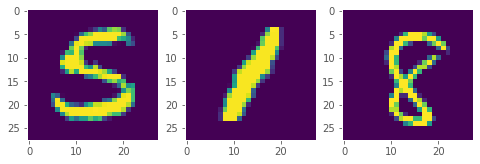``````Bag number: 4
``````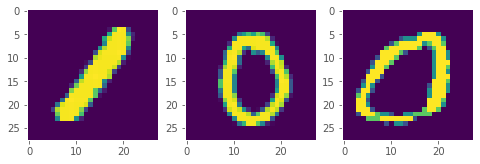``````Bag number: 6
``````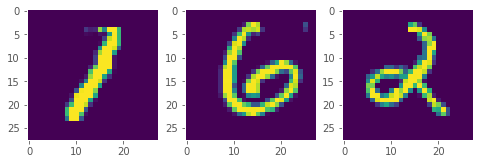``````The bag class label is negative
Bag number: 1
``````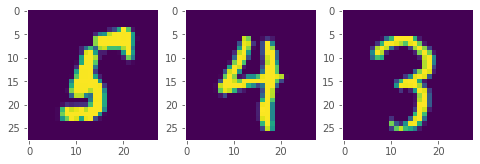``````Bag number: 2
``````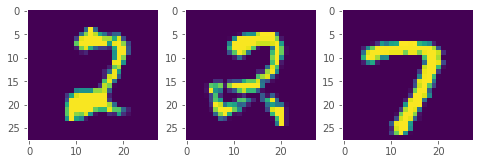``````Bag number: 3
``````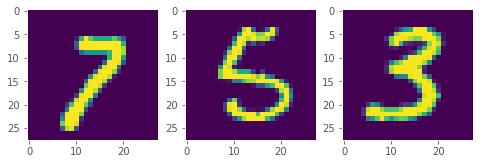## Create model

First we will create some embeddings per instance, invoke the attention operator and then use the softmax function to output the class probabilities.

``````def create_model(instance_shape):

# Extract features from inputs.
inputs, embeddings = [], []
shared_dense_layer_1 = layers.Dense(128, activation="relu")
shared_dense_layer_2 = layers.Dense(64, activation="relu")
for _ in range(BAG_SIZE):
inp = layers.Input(instance_shape)
flatten = layers.Flatten()(inp)
dense_1 = shared_dense_layer_1(flatten)
dense_2 = shared_dense_layer_2(dense_1)
inputs.append(inp)
embeddings.append(dense_2)

# Invoke the attention layer.
alpha = MILAttentionLayer(
weight_params_dim=256,
kernel_regularizer=keras.regularizers.l2(0.01),
use_gated=True,
name="alpha",
)(embeddings)

# Multiply attention weights with the input layers.
multiply_layers = [
layers.multiply([alpha[i], embeddings[i]]) for i in range(len(alpha))
]

# Concatenate layers.
concat = layers.concatenate(multiply_layers, axis=1)

# Classification output node.
output = layers.Dense(2, activation="softmax")(concat)

return keras.Model(inputs, output)
``````

## Class weights

Since this kind of problem could simply turn into imbalanced data classification problem, class weighting should be considered.

Let's say there are 1000 bags. There often could be cases were ~90 % of the bags do not contain any positive label and ~10 % do. Such data can be referred to as Imbalanced data.

Using class weights, the model will tend to give a higher weight to the rare class.

``````def compute_class_weights(labels):

# Count number of postive and negative bags.
negative_count = len(np.where(labels == 0))
positive_count = len(np.where(labels == 1))
total_count = negative_count + positive_count

# Build class weight dictionary.
return {
0: (1 / negative_count) * (total_count / 2),
1: (1 / positive_count) * (total_count / 2),
}
``````

## Build and train model

The model is built and trained in this section.

``````def train(train_data, train_labels, val_data, val_labels, model):

# Train model.
# Prepare callbacks.
# Path where to save best weights.

# Take the file name from the wrapper.
file_path = "/tmp/best_model_weights.h5"

# Initialize model checkpoint callback.
model_checkpoint = keras.callbacks.ModelCheckpoint(
file_path,
monitor="val_loss",
verbose=0,
mode="min",
save_best_only=True,
save_weights_only=True,
)

# Initialize early stopping callback.
# The model performance is monitored across the validation data and stops training
# when the generalization error cease to decrease.
early_stopping = keras.callbacks.EarlyStopping(
monitor="val_loss", patience=10, mode="min"
)

# Compile model.
model.compile(
)

# Fit model.
model.fit(
train_data,
train_labels,
validation_data=(val_data, val_labels),
epochs=20,
class_weight=compute_class_weights(train_labels),
batch_size=1,
callbacks=[early_stopping, model_checkpoint],
verbose=0,
)

return model

# Building model(s).
instance_shape = train_data.shape
models = [create_model(instance_shape) for _ in range(ENSEMBLE_AVG_COUNT)]

# Show single model architecture.
print(models.summary())

# Training model(s).
trained_models = [
train(train_data, train_labels, val_data, val_labels, model)
for model in tqdm(models)
]
``````
``````Model: "model"
__________________________________________________________________________________________________
Layer (type)                   Output Shape         Param #     Connected to
==================================================================================================
input_1 (InputLayer)           [(None, 28, 28)]     0           []

input_2 (InputLayer)           [(None, 28, 28)]     0           []

input_3 (InputLayer)           [(None, 28, 28)]     0           []

flatten (Flatten)              (None, 784)          0           ['input_1']

flatten_1 (Flatten)            (None, 784)          0           ['input_2']

flatten_2 (Flatten)            (None, 784)          0           ['input_3']

dense (Dense)                  (None, 128)          100480      ['flatten',
'flatten_1',
'flatten_2']

dense_1 (Dense)                (None, 64)           8256        ['dense',
'dense',
'dense']

alpha (MILAttentionLayer)      [(None, 1),          33024       ['dense_1',
(None, 1),                       'dense_1',
(None, 1)]                       'dense_1']

multiply (Multiply)            (None, 64)           0           ['alpha',
'dense_1']

multiply_1 (Multiply)          (None, 64)           0           ['alpha',
'dense_1']

multiply_2 (Multiply)          (None, 64)           0           ['alpha',
'dense_1']

concatenate (Concatenate)      (None, 192)          0           ['multiply',
'multiply_1',
'multiply_2']

dense_2 (Dense)                (None, 2)            386         ['concatenate']

==================================================================================================
Total params: 142,146
Trainable params: 142,146
Non-trainable params: 0
__________________________________________________________________________________________________
``````

## Model evaluation

The models are now ready for evaluation. With each model we also create an associated intermediate model to get the weights from the attention layer.

We will compute a prediction for each of our `ENSEMBLE_AVG_COUNT` models, and average them together for our final prediction.

``````def predict(data, labels, trained_models):

# Collect info per model.
models_predictions = []
models_attention_weights = []
models_losses = []
models_accuracies = []

for model in trained_models:

# Predict output classes on data.
predictions = model.predict(data)
models_predictions.append(predictions)

# Create intermediate model to get MIL attention layer weights.
intermediate_model = keras.Model(model.input, model.get_layer("alpha").output)

# Predict MIL attention layer weights.
intermediate_predictions = intermediate_model.predict(data)

attention_weights = np.squeeze(np.swapaxes(intermediate_predictions, 1, 0))
models_attention_weights.append(attention_weights)

loss, accuracy = model.evaluate(data, labels, verbose=0)
models_losses.append(loss)
models_accuracies.append(accuracy)

print(
f"The average loss and accuracy are {np.sum(models_losses, axis=0) / ENSEMBLE_AVG_COUNT:.2f}"
f" and {100 * np.sum(models_accuracies, axis=0) / ENSEMBLE_AVG_COUNT:.2f} % resp."
)

return (
np.sum(models_predictions, axis=0) / ENSEMBLE_AVG_COUNT,
np.sum(models_attention_weights, axis=0) / ENSEMBLE_AVG_COUNT,
)

# Evaluate and predict classes and attention scores on validation data.
class_predictions, attention_params = predict(val_data, val_labels, trained_models)

# Plot some results from our validation data.
plot(
val_data,
val_labels,
"positive",
predictions=class_predictions,
attention_weights=attention_params,
)
plot(
val_data,
val_labels,
"negative",
predictions=class_predictions,
attention_weights=attention_params,
)
``````
``````The average loss and accuracy are 0.00 and 100.00 % resp.
The bag class label is positive
Bag number: 0
``````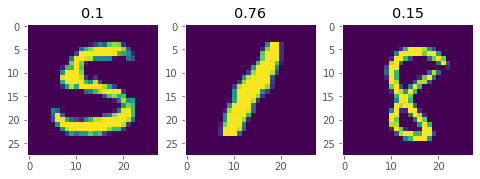``````Bag number: 4
````````````Bag number: 6
``````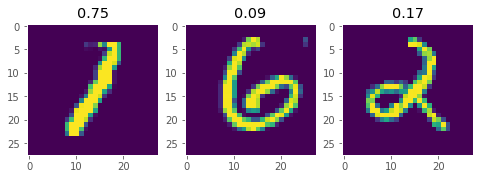``````The bag class label is negative
Bag number: 1
``````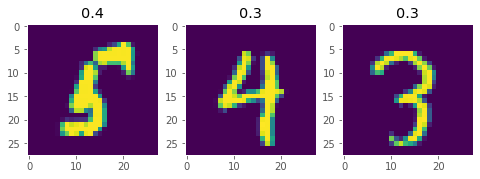``````Bag number: 2
``````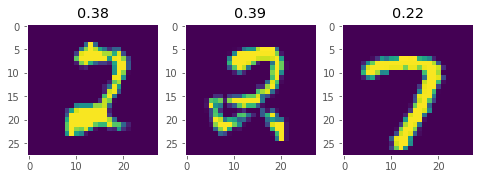``````Bag number: 3
``````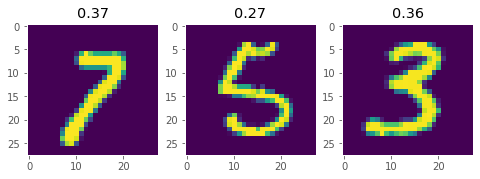## Conclusion

From the above plot, you can notice that the weights always sum to 1. In a positively predict bag, the instance which resulted in the positive labeling will have a substantially higher attention score than the rest of the bag. However, in a negatively predicted bag, there are two cases:

• All instances will have approximately similar scores.
• An instance will have relatively higher score (but not as high as of a positive instance). This is because the feature space of this instance is close to that of the positive instance.

## Remarks

• If the model is overfit, the weights will be equally distributed for all bags. Hence, the regularization techniques are necessary.
• In the paper, the bag sizes can differ from one bag to another. For simplicity, the bag sizes are fixed here.
• In order not to rely on the random initial weights of a single model, averaging ensemble methods should be considered.

Example available on HuggingFace.